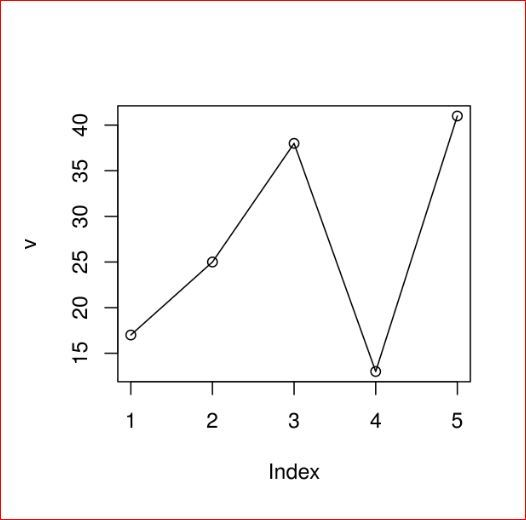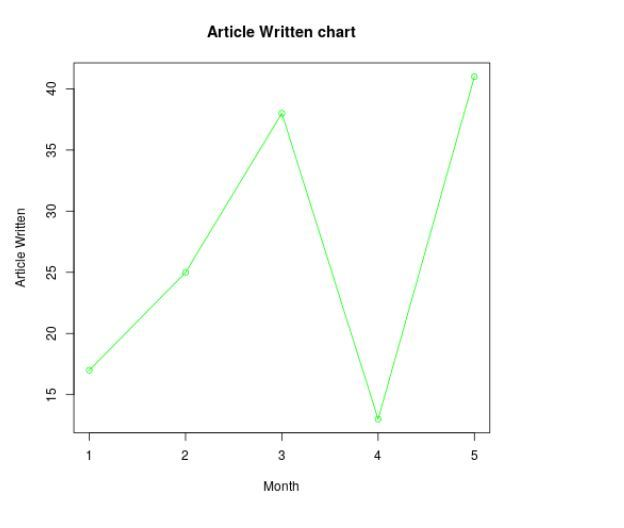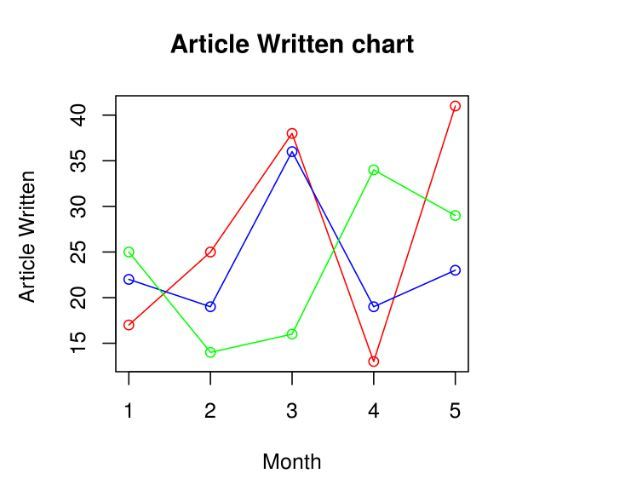# R – Line Graphs

A line graph is chart that is used to display information in the form of series of data points. It utilizes points and lines to represent change over time. Line graphs are drawn by plotting different points on their X coordinates and Y coordinates, then by joining them together through a line from beginning to end. Graph represents different values as it can move up and down based on the suitable variable.

The plot() function in R is used to create the line graph.

Syntax:

`plot(v, type, col, xlab, ylab)`

Parameters:

• v: This parameter is a contains only the numeric values
• type: This parameter has the following value:
1. “p” : This value is used to draw only the points.
2. “l” : This value is used to draw only the lines.
3. “o”: This value is used to draw both points and lines
• xlab: This parameter is the label for x axis in the chart.
• ylab: This parameter is the label for y axis in the chart.
• main: This parameter main is the title of the chart.
• col: This parameter is used to give colors to both the points and lines.

### Creating a Simple Line Graph

Approach: In order to create a line graph:

1. It is created using the type parameter as “o” and input vector.
2. Below code to describe the line graph.

Example:

 `# Create the data for the chart. ` `v <``-` `c(``17``, ``25``, ``38``, ``13``, ``41``) ` ` `  `# Plot the bar chart.  ` `plot(v, ``type` `=` `"o"``) `

Output:### Adding Title, Color and Labels in Line Graph

Approach: To create colored and labeled line chart.

1. Take all parameters which are required to make line chart by giving a title to the chart and add labels to the axes.
2. We can add more features by adding more parameters with more colors to the points and lines.

Example:

 `# Create the data for the chart. ` `v <``-` `c(``17``, ``25``, ``38``, ``13``, ``41``) ` ` `  `# Plot the bar chart. ` `plot(v, ``type` `=` `"o"``, col ``=` `"green"``, ` `     ``xlab ``=` `"Month"``, ylab ``=` `"Article Written"``, ` `     ``main ``=` `"Article Written chart"``) `

Output:### Multiple Lines in a Line Graph

Approach: To create multiple line graphs.

1. In above example, we created line graphs by only one line in each graph.
2. Now creating multiple lines to describe it more clearly.

Example:

 `# Create the data for the chart. ` `v <``-` `c(``17``, ``25``, ``38``, ``13``, ``41``) ` `t <``-` `c(``22``, ``19``, ``36``, ``19``, ``23``) ` `m <``-` `c(``25``, ``14``, ``16``, ``34``, ``29``) ` `  `  `# Plot the bar chart. ` `plot(v, ``type` `=` `"o"``, col ``=` `"red"``,  ` `     ``xlab ``=` `"Month"``, ylab ``=` `"Article Written "``,  ` `     ``main ``=` `"Article Written chart"``) ` `  `  `lines(t, ``type` `=` `"o"``, col ``=` `"blue"``) ` `lines(m, ``type` `=` `"o"``, col ``=` `"green"``) `

Output: When we execute the above code, it shows the following result-My Personal Notes arrow_drop_upIf you like GeeksforGeeks and would like to contribute, you can also write an article using contribute.geeksforgeeks.org or mail your article to contribute@geeksforgeeks.org. See your article appearing on the GeeksforGeeks main page and help other Geeks.

Please Improve this article if you find anything incorrect by clicking on the "Improve Article" button below.

Article Tags :

Be the First to upvote.

Please write to us at contribute@geeksforgeeks.org to report any issue with the above content.